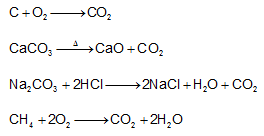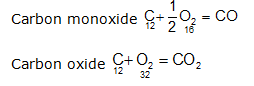Laws of Chemical Combination

Laws of Chemical Combination- In order to understand the composition of the compounds, it is necessary to have a theory which accounts for both qualitative and quantitative observations during chemical changes. Observations of chemical reactions were most significant in the development of a satisfactory theory of the nature of matter. These observations of chemical reactions are summarized in certain statements known as laws of chemical combination. These are:

1-Law of conservation of mass

2-Law of constant composition

3-Law of multiple proportions

4-Law of reciprocal proportion

5-Law of combining volumes (Gay Lussac's law of gaseous volume)

1-Law of conservation of mass-This law deals with the masses of reactant & the products of any chemical reaction (or a physical change).It was studied by French chemist Antoine Lavoisier. This law may be stated as follow-In all the chemical & physical changes, the total mass of the reactants is equal to that of the products. . It is a derivation of Dalton's atomic theory 'atoms neither created nor destroyed'.

Total masses of [reactants=products+unreacted reactants]

For example-1-A piece of ice in flask (weighted) is heated gently to melt ice (solid) into water (liquid) & again weighted. It's found that there is no change in weight though a physical change has taken place.

2-If 5.2 g of CaCo2when heated produced 1.99 g of Carbon dioxide and the residue (CaO) left behind weighs 3.2g.

So the total weight of the products (CaO +CO2) = 3.20+ 1.99 = 5.19 g

Difference between the wt. of the reactant and the total wt. of the products= 5.20 - 5.19 =0.01 g.

This small difference may be due to experimental error. Thus law of conservation of mass holds good with in experimental errors.

2-Law of constant composition or the definite proportions-This law was given by J.L.Proust & deals with the composition of elements present in a given compound. It states that- A chemical compound always contains same elements combined together in same proportion by mass. and it does not depend on the source of compound. For example-CO2 can be obtained by different method such asThe composition of CO2 obtained by different means always having same C: O ratio =12/32 = 0.375 by mass.

Limitations of law of constant composition are-

(i)This law is not applicable if an element exists in different isotopes which may be involved in formation of compound.e.g.In the formation of CO2, if C-12 isotope & C-14 isotope combine than the C: O is 12:32 & 14:32 respectively.

(ii)The compounds formed may be different but the elements may combine in same ratio.

3-Law of multiple proportion: It was studied by Dalton, which defined as follow-

When two elements combine to form two or more than two different chemical compounds then the different masses of one element which combine with fixed mass of the other element bear a simple ratio to one another.

For example: Carbon forms two oxides in oxygenThe ratio of masses of oxygen in CO and CO2 for fixed mass of carbon (12)
is 16 : 32 = 1: 2.

Similar with compounds of sulphur & oxygen-they also formed two oxides SO2 & SO3 so the ratio of masses of oxygen 32:48 or 2:3 respectively.

4-Law of reciprocal proportion: This law was given by Richter. It stated as follow-

If two elements combine separately with a fixed mass of a third element, then the ratio of their masses in which they do so is either same or multiple of the ratio in which they combine with each other. The above law is the basis of law of equivalent masses.

5-Law of combining volumes (Gay Lussac's law of gaseous volume) - It states that at a given temperature and pressure, when the gases combine they do so in volumes which bear a simple ratio to each other and also to the volume of gaseous product.

e.g. If one volume of hydrogen react with one volume of chlorine to form two volumes of hydrogen chloride gas. The ratio of volume of various reactant & product is 1:1:2 which is a simple whole number ratio. Similar in the formation of ammonia, the ratio of reactant nitrogen, hydrogen & ammonia is 1:3:2 which is a simple whole number ratio.

#### Related Questions in Chemistry

• ##### Q :Concentration of urea Help me to go

Help me to go through this problem. 6.02x 1020 molecules of urea are present in 100 ml of its solution. The concentration of urea solution is: (a) 0.02 M (b) 0.01 M (c) 0.001 M (d) 0.1 M (Avogadro constant, N4= 6.02x 1023mol -1)<

• ##### Q :Group IV Cations Chromium(III)

Chromium(III) hydroxide is highly insoluble in distilled water but dissolves readily in either acidic or basic solution. Briefly explain why the compound can dissolve in acidic or in basic but not in neutral solution. Write appropriate equations to support your answer.

• ##### Q :6. 20 gm of hydrogen is present in 5

6. 20 gm of hydrogen is present in 5 litre vessel. The molar concentration of hydrogen is

• ##### Q :Calculating density of water using

What is the percent error in calculating the density of water using the ideal gas law for the following conditions:  a. 110 oC, 1 bar   b. 210 oC 10 bar  c. 374 o

• ##### Q :Vant Hoff factor The Van't Hoff factor

The Van't Hoff factor of the compound K3Fe(CN)6 is: (a) 1  (b) 2  (c) 3  (d) 4  Answer: (d) K3[Fe(CN)6] → 3K+

• ##### Q :Concentration of Sodium chloride

Provide solution of this question. If 25 ml of 0.25 M NaCl solution is diluted with water to a volume of 500ml the new concentration of the solution is : (a) 0.167 M (b) 0.0125 M (c) 0.833 M (d) 0.0167 M

• ##### Q :Mole fraction Give me answer of

Give me answer of following question. The sum of the mole fraction of the components of a solution is : (a) 0 (b) 1 (c) 2 (d) 4.

• ##### Q :Relative lowering of the vapour pressure

Choose the right answer from following.The relative lowering of the vapour pressure is equal to the ratio between the number of: (a) Solute moleules and solvent molecules (b) Solute molecules and the total molecules in the solution (c) Solvent molecules and the tota

• ##### Q :Extensive property Choose the right

Choose the right answer from following. Which one of the following is an extensive property: (a) Molar volume (b) Molarity (c) Number of moles (d) Mole fraction

• ##### Q :Question based on vapour pressure and

Benzene and toluene form nearly ideal solutions. At 20°C, the vapour pressure of benzene is 75 torr and that of toluene is 22 torr. The parial vapour pressure of benzene at 20°C for a solution containing 78g of benzene and 46g of toluene in torr is: (a) 50 (b)